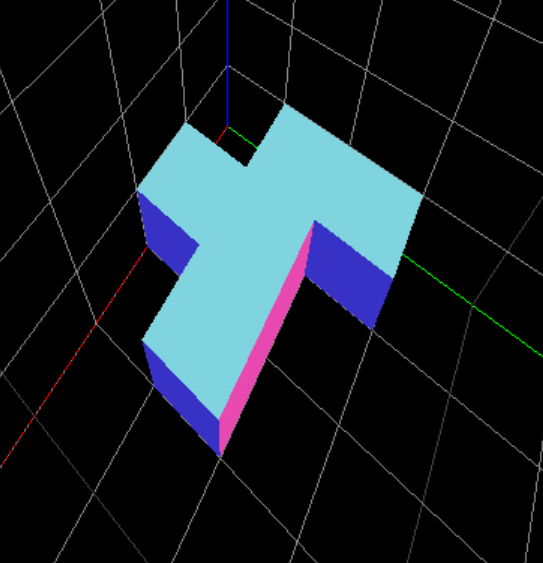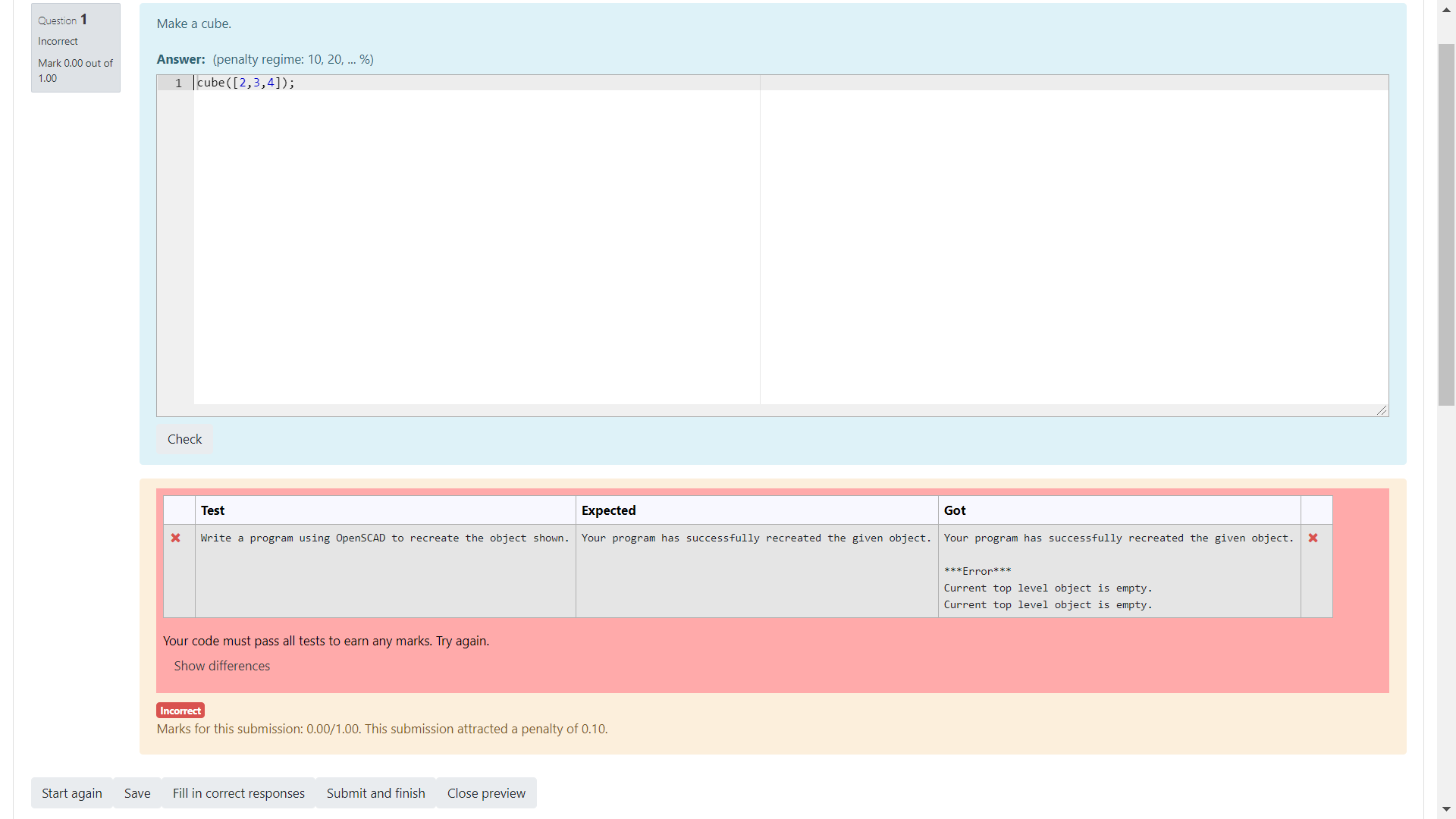## Question Authors' Forumby Michael Backus -
Number of replies: 5

I'm working on a new question type using OpenSCAD. I'd like to ask students to create a 3D object using OpenSCAD and have them be able to submit any solution that successfully recreates the object. Here's an example:Below are two possible and equally valid solutions.

Solution A:

translate([0,10,0])
cube([10,20,10]);
translate([10,0,0])
cube([10,20,10]);
translate([20,10,0])
cube([10,10,10]);

Solution B:

translate([10,0,0])
cube([10,10,10]);
translate([0,10,0])
cube([30,10,10]);
translate([0,20,0])
cube([10,10,10]);

I hope to do this by first verifying the student's solution is valid code (test 1), then subtracting the student solution from a correct solution and looking for a "Current top level object is empty." error (test 2), and then subtracting the correct solution from the student solution and again looking for a "Current top level object is empty." (test 3). If I receive those two errors, then I can conclude that the shapes are a perfect match.

I've done this by creating an OpenSCAD via python question prototype. Using

subprocess.call(["/home/pi/.local/bin/openscad","-o","prog.stl","prog.scad"])

I am able to perform test 1.  If the output is 0, then the code is valid.

Using

student_answer = """difference() {\n {{ STUDENT_ANSWER }}\n{{ QUESTION.answer }} }"""

test2 = subprocess.call(["/home/pi/.local/bin/openscad","-o","prog.stl","prog.scad"])

I am able to perform test 2. If the output is 1 then there was an error, which is what I want in this case. I use similar code to perform test 3, again looking for an error. I created a dummy question that shows the Expected and Got boxes are the same, except the Got box includes the error messages, which I think is causing the answer to be graded as incorrect.I'm not sure how to solve this problem. Any help would be greatly appreciated. I've attached the question prototype and the dummy question for your reference.by Richard Lobb -

I think the problem is that the error messages are going to stderr and CodeRunner interprets stderr output as a runtime error. This results in the ***Error*** line followed by the collected stderr output.

You should be able to fix it just by adding an argument stderr=subprocess.DEVNULL to your subprocess.call calls.by Michael Backus -

Your suggestion worked, but now I'm facing a new problem. I tried grading a model that involves a sphere which takes OpenSCAD some time to render. I get a timeout error. I can think of two ways to handle this:

1. Increase the Jobe server timeout (I don't like this solution).
2. Run all three tests at once. I'm able to do this from the command line easily using the & operator to combine commands, but I'm not sure how to do the same thing with CodeRunner. Is this possible? If so, what's the best way to combine my tests so they run concurrently and take less time?by Richard Lobb -

I don't have a simple solution here. But I'm a bit horrified that you can't model a sphere in less than a few seconds. Is there no way to crank down the resolution?

If a timeout occurs when you attempt to run all tests in a single run (i.e., a combinator template), the normal built-in CodeRunner question types all drop back to running tests one by one, each as a separate Jobe submission. So if you still get to see a timeout in the result table it means that that test-case in isolation timed out. Running all tests in parallel on different cores won't help, except that the student will get their feedback faster.by Michael Backus -

I can reduce the resolution by rendering circles with 12 sides, but I'm concerned that some of the future more complicated questions I wish to pose will cause a timeout.

I see now that using the term "test" was confusing. Within my template I conduct three different tests to see if the student's answer was a match. Let's call these developer tests, to distinguish them from the tests that a question author poses. Here's my code:

""" The template for a question type that compiles and runs a student-submitted
"""

import subprocess, sys

# Test #1

# Test 2

# Test 3

if test1 == 0 and test2 == 1 and test3 == 1:
print("Match")
elif test1 == 1:
print("Error")
elif test2 == 0 or test3 == 0:
print("Mismatch")


I'm guessing there's a way to use a subprocess.call to run all three developer tests at once. When I do this from the command line using the "&" character to join commands for these tests, it makes a significant difference with respect to the amount of time taken. Just want to figure out how to get the template to do something similar. Any ideas?